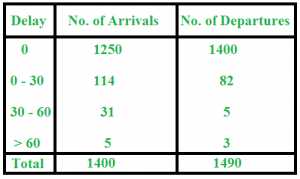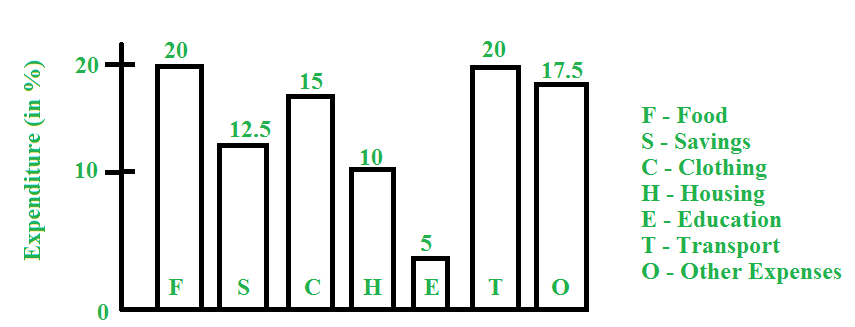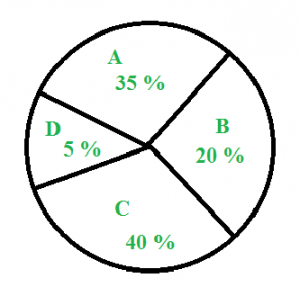Open in App
Not now

# Data Interpretation

• Difficulty Level : Easy
• Last Updated : 28 Jun, 2021

In data interpretation, we are given data in the form of visuals, like table, graph, chart, etc. and a set of questions that follow. Mainly, they use percentage , ratio or proportion.
Here, we discuss some commonly asked questions.

### Tables

Question : The following table contains the data of train arrival and train departure from a station. Study the table and answer the questions that follow :a) Find the number of trains that arrived late
b) Find the number of trains that departed late
c) Find the percentage of late arriving trains
Solution :
a) Number of trains arriving late = 114 + 31 + 5 = 150
b) Number of trains departing late = 82 + 5 + 3 = 90
c) Percentage of late arriving trains = (150 / 1400) x 100 = 10.71 %

### Bar Graph

Question : Study the following bar graph and answer the questions that follow :
Total monthly income = Rs. 50,000a) What amount is spent on food ?
b) How much more money is spent on clothing and housing together than on transportation?
c) What percent of amount on food is spent as amount on education?
Solution :
a) Food = 20 % of expenditure
=> Amount spent on food = 20% of 50,000 = Rs. 10,000
b) Money spent on clothing and housing together = 25 % of Rs. 50,000 = Rs. 12,500
Money spent on transportation = 20 % of Rs. 50,000 = Rs. 10,000
Therefore, more money spent on clothing and housing together than on transportation = Rs. 12,500-10,000 = Rs. 2,500
c) Expenditure on food = 20 %
Expenditure on education = 5 %
Therefore, percent of amount on food spent as amount on education = (5 / 20) x 100 = 25 %

### Pie Chart

Question : Study the pie chart below and answer the questions that follow :The above pie chart shows the sales of four different types of articles in a shop.
a) What is the central angle of type A ?
b) If the total sale is 1200, what is the sale of B ?
c) What is the difference between the central angle of C and D ?
Solution :
a) Central angle of A = Percentage of A x 360 degrees = (35 / 100) x 360 = 126 degrees
b) Sales of B = 20 % of 1200 = 240
c) Difference between the central angle of C and D = 40 % of 360 – 5 % of 360 = 35 % of 360 = 126 degrees